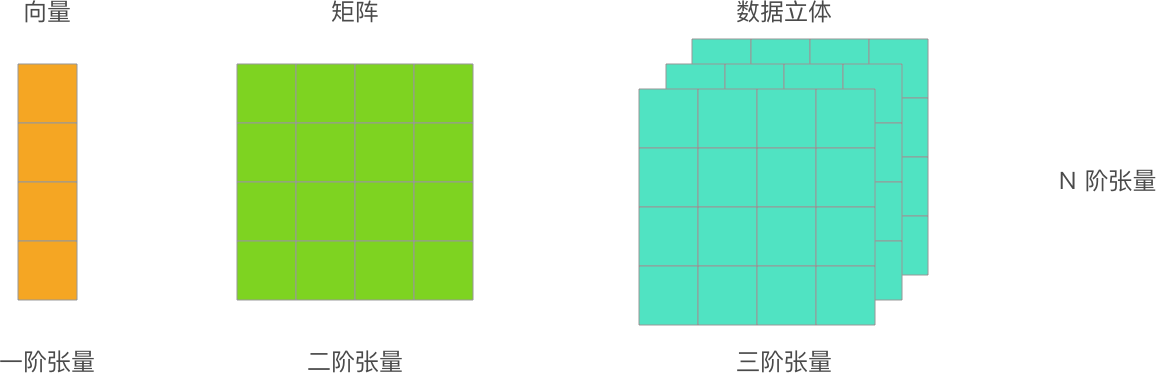机器学习常见术语表

D

• 一个元素设为 1。
• 所有其他元素均设为 0。

One-Hot 编码常用于表示拥有有限个可能值的字符串或标识符。例如，假设某个指定的植物学数据集记录了 15000 个不同的物种，其中每个物种都用独一无二的字符串标识符来表示。在特征工程过程中，您可能需要将这些字符串标识符编码为 One-Hot 向量，向量的大小为 15000。

J

• 不同的初始化
• 不同的超参数
• 不同的整体结构$$召回率 = \frac { 真正例数 } { 真正例数 + 假负例数 }$$

$$H(p,q) = \sum_{i=1}^{n} p(x) \cdot log(\frac{1}{q(x)})$$

• 期望构建最具预测性的模型（例如损失最低）。
• 期望使模型尽可能简单（例如强大的正则化）。

L

• 绝对值很高的权重。
• 与实际值相差很大的预测值。
• 值比平均值高大约 3 个标准偏差的输入数据。

P

$$y' = b + w_1x_1 + w_2x_2 + … w_nx_n$$

R

ROC 曲线下面积的数值意义为：对于随机选择的正类别样本确实为正类别，以及随机选择的负类样本为正类别，分类器更确信前者的概率。

S

SGD 依赖于从数据集中随机均匀选择的单个样本来计算每步的梯度估算值。

• L2 损失函数
• 对数损失函数
• L1 正则化
• L2 正则化

Z

$$准确率 = \frac { 正确预测数 } { 样本总数 }$$

$$准确率 = \frac { 真正例数 + 真负例数 } { 样本总数 }$$

$$召回率 = \frac { 真正例数 } { 真正例数 + 假正例数}$$

TensorFlow 程序中的主要数据结构。张量是 N 维（其中 N 可能非常大）数据结构，最常见的是标量、向量或矩阵。张量的元素可以包含整数值、浮点值或字符串值。